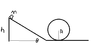# Circular Motion Loop question

• squirrelschaser
In summary, the problem involves a child and a cart starting at rest on a frictionless ramp and entering a frictionless circular loop. The question is asking for the minimum height of the ramp in order for the cart to successfully traverse the loop. The incorrect solution attempted to use conservation of energy, while the correct solution uses kinematics and calculates the required height to be half the radius of the loop. The provided solution may be incorrect and there may be additional information needed to solve the problem using kinematics.

## Homework Statement

Bob starts at rest from the top of a frictionless ramp. At the bottom of the ramp, he enters a frictionless circular loop. The total mass of the child and the cart he sits in his m. What must the height of the ramp be in order for the cart to successfully traverse the loop.

h = height of ramp
theta = angle of the ramp (irrelevant though)

## The Attempt at a Solution

[/B]
I solved for the minimum speed at the top of the loop.

Fy = F + mg = mv^2/r

v= sqrt(rg)

I then used conservation of energy.

Initial : mgh
Final : mg2r + (m(sqrt(rg))^2)/2

mgh = mg2r + mrg/2

mgh = 5mgr/2

Cancel stuff out h = 5r/2 (WRONG)

Instead the solution calls for using kinematics not energy conservation.

v= sqrt(rg) stills hold.

vf^2 = vi^2 + 2ax

rg = 0 + 2gsin(theta)*(h/sin(theta)

rg = 2gh

r = 2h

I understand the mathematical process of the correct solution.
However, I don't understand why I can't use conservation of energy(gives me wrong answer) instead of kinematics.

#### Attachments

•help.png
2 KB · Views: 387
The second answer (r/2) is clearly wrong since it would not provide enough energy to reach the top of the loop even with no remaining KE.
The calculation goes wrong because it equates the speed at the bottom of the ramp to that required at the top of the loop.

The solutions I have showed the second answer as the correct answer.
haruspex said:
The second answer (r/2) is clearly wrong since it would not provide enough energy to reach the top of the loop even with no remaining KE.
The calculation goes wrong because it equates the speed at the bottom of the ramp to that required at the top of the loop.

Really? That's the solution provided to me.
Glad to know I wasn't paranoid or something.

Is there any additional information that would make solving this question using kinematic possible, then?

squirrelschaser said:
Is there any additional information that would make solving this question using kinematic possible, then?
The v2-u2=2as equation is effectively KE+PE constant. All that's different is factoring out the mass.